# Core Engineering Language (C-EKL)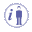Note that this language inherits the components of the Mathematical Engineering Language (M-EKL).

C-EKL adds the following language elements:

• Keywords for control structures, like the "if... then... else" conditional statement in rules.
• Specific functions, like the ones dedicated to messages and prompts for user inputs, geometry construction or strings and lists manipulation.
• Additional operators, like the "=>" operator, which corresponds to a kind of "imply" keyword for checks.

C-EKL is used in the following Knowledge artifacts that are related to the update process through their parameters: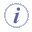Rules, Checks, formula and design tables are objects integrated to the update process of CATIA V5. To be compliant with the update process, it is important that those objects manipulate only parameters as inputs or outputs (parameters being simple values, lists or geometric datums). It is recommended not to use them to access attributes of those objects nor call methods.

## Knowledgeware and Extended Functions

 Design tables Keywords Law List Mathematical Functions Messages Operators String Analysis Operators Circle Constructors Direction Constructors Electrical Functions Line Constructors Measures Part Measures Plane Constructors Point Constructors Surface Constructors Wireframe Constructors
 Operators belonging to Knowledge products. Operators and constructors belonging to non Knowledge products.The information provided below constitute the ground knowledge you must know to work with C-EKL.

## Control Structures

### Conditional Statements

#### Rules

if  ... else  ... else if
Conditionally executes a group of statements, depending on the value of an expression. You can use either block form syntaxes:
 ``if`` condition statements [else elsestatements ]`` `or` ````if```` condition      { statements } [else if condition-n       [  { elseifstatements  } ] ] . . . [else     [  { elsestatements } ] ]``````
You can use the single-line form (first syntax) for short, simple rules. However, the block form (second syntax) provides more structure and flexibility than the single-line form and is usually easier to read, maintain, and test.
The else and else if clauses are both optional. You can have as many else if statements as you want below a block if, but none can appear after the else clause. Block if statements can be nested that is, contained within one another.

#### Checks

Checks can only read parameters. As a consequence, you cannot use functions that have arguments in output.

 ``Relations\Formula.1\Activity == false``

Checks can use a specific keyword =>. statement1  => statement2  (if statement1  then statement2).
Displays a message (if type is Warning or Information) and turns to red in the specification tree each time statement2 is invalid as statement1 is fulfilled.

 OK => KO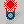KO => KO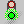KO => OKOK => OK### For Statement

The first usage of the For keyword is a loop based on the element of a list. See syntax opposite.

Where:

• x is a variable name (of a given type. It may represent an object or a value).
• x can be used in the body (like any other variable of the language). It contains the element of the list corresponding to the current iteration.
• List is a variable name of type List or an expression returning a list.

The body is executed Nth times where N is the number of elements of the list.

```let List.1(List) let x(Point) For x inside List { … Body … if (x <> NULL) }```
The second usage of the For keyword executes a loop until an expression becomes false. See syntax opposite.

Where:

• x is a variable name of integer type. It is incremented at the end of each execution of the body.
• predicate is a Boolean expression. The body is executed if this expression is true. This expression is evaluated before the body.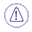Note that the second usage of the For operator can lead to infinite loops.
```For x while predicate { … Body … }```

### While Statement

 This loop executes until an expression becomes false. See syntax opposite.Where: i is a variable name of integer type. It is incremented at the end of each execution of the body. X is a variable for points. ```let i = 1 let x(Point) for i while i<=parameter.Size() { x = parameter.GetItem(i) if (x.GetAttributeReal("Y") < 0.04) x.SetAttributeReal("Y",0.04) }```

### Definitions

A function is characterized by its signature, its input arguments and its output argument.
A method is associated to an object. It is characterized by its signature that contains # in and # out.

### Working with Variables

#### Passing Variables By Reference

The Knowledgeware language passes variables systematically by reference when calling a function. Assigning by reference means that the new variable references (in other words, "points to") the original variable. Changes to the new variable affect the original, and vice versa. This also means that no copying is performed. Therefore, when a function or a method is called, no value is copied. Arguments are passed by reference. If you write `sin(x)`, the variable x will be directly available in the body of the sinus function.

#### Strong Typing

The Knowledgeware language is strongly typed meaning that certain rules must be respected.

##### Variables Passed as Argument

A variable passed as argument (in input) of a function must be at least a sub-type of this function argument or their types must be similar. If you write `area (s)`, `s` must be a surface. This is also true for the return argument of a function. For more information about the hierarchy of types, see Advanced Engineering Language.Some functions (like `list>GetItem`) return objects of undetermined type. When the Undetermined type is the result of a method or function, use a (local) variable. Calling 2 functions, one of them resulting in an Undetermined type, may be confusing when this function is overloaded.
 Example: `a = intersect (List > GetItem(1),Surface.1)`

In the above example, you do not know if you make an intersection between 2 surfaces or between a curve and a surface. It is therefore highly recommended to write:

 ```let x (Curve) x = List > GetItem(1) a = intersect (x,Surface.1)```
##### Affectation Rules

When setting a variable, the type of the value must be a sub-type of the variable creation type. It is impossible to change the type of a variable after its creation.

Note that there are 4 exceptions:

• The set keyword enables you to affect a variable without checking the type. If the object of the member located at the right of the affectation also supports the type of the variable located at the left, the affectation is performed. If not, the variable is set to NULL.
###### Example 1:

`set x=y`, `x` being a variable of Point type and `y` a variable of Solid type. `x=NULL`

###### Example 2:

If you type:

 ```let C1(Curve) let C2(Curve) C1 = intersect(PartBody\Extrude.1, `xy plane` ) C2 = intersect(PartBody\Extrude.2 ,`xy plane` ) PartBody\Line.2 = C1 PartBody\Line.3 = C2```

The affectation does not work since you are trying to affect a line to a curve.

If you type:

 ```let C1(Curve) let C2(Curve) C1 = intersect(PartBody\Extrude.1, `xy plane` ) C2 = intersect(PartBody\Extrude.2 ,`xy plane` ) set PartBody\Line.2 = C1 set PartBody\Line.3 = C2```

and if C1 and C2 are of line type, the affectation is performed.

• The NULL keyword is a constant. When this constant is passed as argument of a function, no check is performed on the type thus enabling you to set any variable to NULL or to compare a variable with NULL to find out if it is unset.
 `pointOncurve (C, 10mm, NULL)`
• The return type of some methods is UndeterminedType. In such a case, you can valuate any variable with this function return.
 `x = List>GetItem`
• When working with variables of Value type, no check is performed on the real type variables, thus enabling you to:
 Valuate a real with an integer Valuate a real with an angle Valuate a length with a real Valuate a length with an angle

###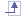#### Management of Local Variables

Local variables can be declared  by using the let keyword. A temporary variable does not persist as a parameter after the rule execution is finished. You can use local variables in 2 different situations:

• The local variable is a parameter (literals, lists, isolated wireframe geometry). In this case, the local variable is a value.
 ``````let x (type) x = parameter```` ````

In this case, the parameter value is affected to x. If you modify x, the parameter value will not be modified.

 ``````let x (real) x = Real.1 x = 0.5 /*Real.1 is not modified````*/ ````
• In all other cases, the local variable has the behavior of a pointer. The type has to be indicated.
 ``````let H(Hole) H= Hole.1 H.Diameter = 20mm ````/*Hole.1 is modified``*/ ````
• There is one exception: When you valuate a local parameter of type datum with a datum in the tree :
 ```let p (Point) p = OpenBody.1\Point.1 /*p becomes the datum, to be able to write then:*/ p = /*Point.1 has been modified*/```

Note that local variables must be declared at the beginning of the rule, before any other instruction is specified.

 ```let S1(Surface) let S2(Surface) let S3(Surface) ``` ```S1 = Split ... S2 = ... S3 = ...```

### Different Ways To...

#### Write a Value

Given p a parameter of Length type.

 ```let p1 = 12mm let p2 = 7 mm let x = 3mm let pt (Point) ``` `x=p` // The variable x is valuated by the value of p `x=p+3` // p is read `pt.coord (p,p1,p2)` // p is valuated by a function with an output argumentSuch a parameter has the same behavior as a local variable.

###### Write an Input Object

Given H1, a Hole (Hole.1)

 ```let x = 0 mm let H3 (Hole) let H4 (Hole) H1.Diameter = 3mm``` // The attribute is valuated``` X=H1.Diameter+3mm``` // The attribute is read ``` H3=H1``` // H3 points to Hole.1``` H4=H3 ```// The H4 variable points to the feature pointed to by H3Such an object is handled like a constant variable. A local variable of the same type has a very similar behavior.

###### Write an Output Object: Datum

Given D1 and D2, 2 existing geometrical datums.

 `let S1 (Surface)` `let S2 (Surface)` `let P1(Point)` `S1 = S2` // The S1 variable points to the feature pointed by the S2 variable `S1 = D1` // The S1 variable points to the datum feature D1 `D1 = D2` // Copies the geometrical result of D2 into D1 `D1 = S1` // Copies the result of the feature pointed by S into D1 `a = area(D1)` // passed as input argument `D1 = point (0mm,0mm,0mm)` // Copies the geometrical result of point into D1 `P1 = point (0mm, 0mm, 0mm) `// The S variable points to the local feature which is the result of the point operator.It is impossible to create local variables of datums but datums can be used through Knowledge types.

###### Write an Output Object: List

Given 2 lists, L1 and L2

 `let L3(List)` `L1.Size() `// L1 as input `L1=L2` // The content is affected `L3=L1` // The content is affected